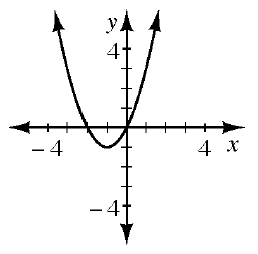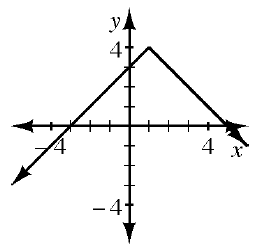### Home > GB8I > Chapter 11 Unit 12 > Lesson INT1: 11.2.1 > Problem11-52

11-52.

For each relation represented below, state the $x$- and $y$-intercepts.

1.Where does the relation intersect the $y$-axis? The $x$-axis? Does it intersect with the same axis multiple times?

1.$y$-intercept: $(0, 3)$
$x$-intercepts: $(3, 0), (5, 0)$

1.  $x$ $y$ $-3$ $-2$ $-1$ $0$ $1$ $2$ $3$ $8$ $3$ $0$ $-1$ $0$ $3$ $8$

Look for the zeros in the table.